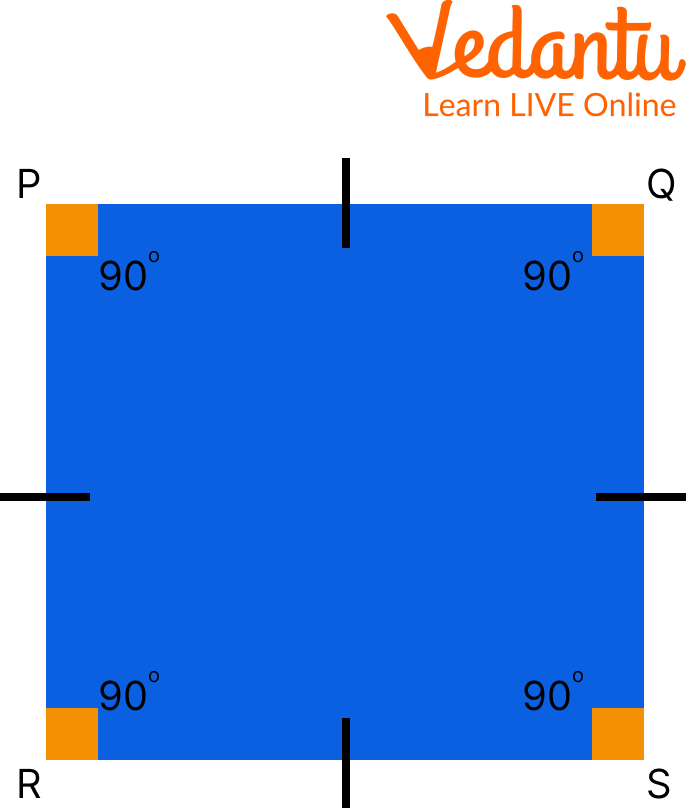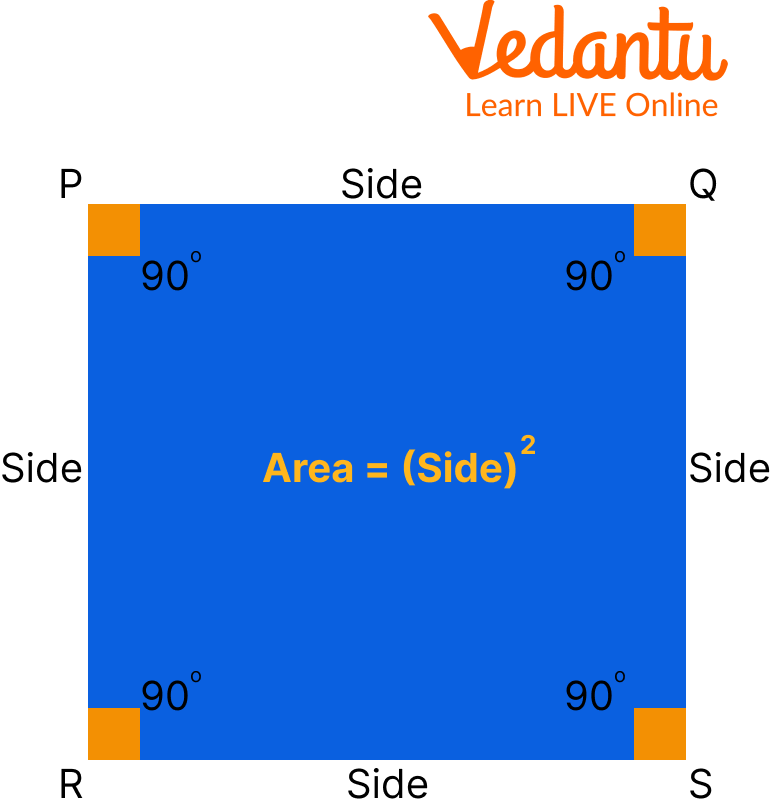Courses
Courses for Kids
Free study material
Free LIVE classes
More

# Dimensions of a SquareLIVE
Join Vedantu’s FREE Mastercalss

Have you ever wondered what a square is or how it looks? Squares are a shape with four equal sides and angles, where the space contained within them is all of the same sizes. The term "square" is a geometric shape that can be used to describe any rectangle or rhombus. Squares are easy to draw and are a common shape in our environment. The dimensions of a square are its length and width. Here you will find the dimensions of a square, you will learn about square sides and how to calculate the area of a square.Picture of chess

## Square Sides

A square is a four-sided closed two-dimensional (2D) shape. A square's four sides are all equal and parallel to one another. The basic shape of a square is depicted below. So, here the question arises, how many sides square have? From the below figure, it is clear now that there is a total of 4 sides in a square. Square sides are as follows: PQ, QS, SR, RP.Square

## What is the Area of Square?

A square's area is the amount of space it takes up. Chessboards, square wall clocks, and other square shapes are examples. The space occupied by these things can be calculated using the area of a square formula. In the below heading we will learn about how to calculate the area of a square.

## How to Calculate Area of a Square?

To find the area of a square, first, calculate one side of the square (all sides of the square are equal ). then multiply by itself to get the area of the square. Hence, $\text { Area }=(\text { Side })^{2}$Area of Square

Similarly, we may calculate the area of any other form based on its sides, such as a rectangle, parallelogram, triangle, or polygon.

Example 1. Calculate the area of the square whose length of square sides is 20m.

Ans: Given, length of square sides(a) = 20m

The area of the square is given by: $a \times a=a^{2}$

$\text { Area }=20 \mathrm{~m} \times 20 \mathrm{~m}=400 \mathrm{~m}^{2}$.

Example 2. Find the area of the square whose length of diagonals is 10 cm.

Ans: First we find the side length of square(a)

Length of diagonal is given by formula = d

Diagonal of square $(\mathrm{d})=\sqrt{ 2}a$

Length of diagonal $=10 \mathrm{~cm}$

Now, Area of the square $=\mathrm{a}=\dfrac{(\mathrm{d})^{2}}{2}$

$\Rightarrow \dfrac{(\mathrm{d})^{2}}{2}=\dfrac{100}{2} =50\mathrm{~cm}^{2}$

Example 3. Calculate the area of a square whose side is $11 \mathrm{~cm}$.

Ans: Given that, side $=11 \mathrm{~cm}$

Area of square is given by $=11 \times 11=121 \mathrm{~cm}^{2}$

## Dimensions of a Square

To determine the dimensions of a square, its area or perimeter must be specified.

Let us assume a square has a surface area of 36 square feet. First, write down the square area Formula: A = x2, where "A" represents the area and "x" represents one of the side lengths. Because the square has four equal sides, you only need to find one dimension. Work out the area equation. It will appear as 36 = x2. To find the dimension of the square, you must first isolate "x", Take the square root of 36 to cancel out the square sign on the right side of the equation. The answer to the square root problem is 6 . The final answer is x = 6, which means that each dimension of the square is 6 m.

Using the perimeter, calculate the dimensions of the square. The perimeter of the square in this case will be 20 m. Write the perimeter equation for a square down: P = 4t, where "P" represents the perimeter and "x" represents the side dimension. Work out the perimeter equation. It will seem as follows: 20 = 5x. Divide each side of the equation by 4 , and write down the result: x = 4. The final result is x = 5, indicating that the square dimensions are 4 m each.

## Practice Questions

Q 1. Find the area of the square whose side is $14 \mathrm{~m}$.

Ans: $196 \mathrm{~m}^{2}$.

Q 2. Find the side of the square whose area is $225 \mathrm{~m}^{2}$.

Ans: $15 \mathrm{~m}$.

## Summary

A square is a plane figure with four equal straight sides and four right angles. It is also a regular polygon, meaning that all of its sides and angles are equal. The area of a square is the space inside the perimeter or boundary of the square. The dimensions of a square are its length and width, which are always the same. We have also learned about the area of square, i.e. Area = (Side)2. In the end we have learned to find the dimensions of the square, using the perimeter and area of the square.

Last updated date: 18th Sep 2023
Total views: 114k
Views today: 3.14k

## FAQs on Dimensions of a Square

1. What are the measuring units for the area and perimeter of the square?

The square has a two-dimensional surface. As a result, the area of a square is always represented by square units, which are commonly represented by cm2, m2, in2, or ft2. A square's perimeter is the sum of its four sides or the length of its boundary, which is a one-dimensional measurement with linear units represented by cm, m, in, or ft.

2. What is the distinction between a square's perimeter and area?

The square has a two-dimensional surface. A square's perimeter is the sum of its four sides or the length of its boundary i.e. s + s + s + s or 4s. Here, "s" is the length of the side of the square. It is a one-dimensional measurement with linear units represented by cm, m, in, or ft. The area of a square is the amount of space occupied by the square in two dimensions. Area = (Side)2. It is measured in square units represented by cm2, m2, in2, or ft2.

3. What is diagonal?

A diagonal in geometry is a line segment that connects two polygonal or polyhedral vertices when they are not on the same edge. Any sloping line is known as a diagonal informally. The diagonal of a square can be defined as a line segment connecting two opposed vertices of the square. Because a square has four vertices, So it has two diagonals. The diagonal length of a square is always greater than the length of its sides.

The area of a square is $=\mathrm{a}=\dfrac{(\mathrm{d})^{2}}{2}$

when a diagonal is given. Similarly, diagonals can be used to determine the perimeter of a square.